# Longitude and Latitude

### Map Location Systems

One of the main purposes of maps is to identify the location of places or features on the surface of the Earth. It is equally important to be able to locate the position of one map relative to another map on the Earth's surface. There are a number of methods of doing this. Most map location systems use some type of regularly spaced lines which form a grid pattern. In some cases these grid lines are parallel, in other cases they converge and meet at a single point. The U.S. Army uses the Universal Transverse Mercator system for its maps and field operations. This system is a rectangular grid based on one square kilometer. The most commonly used location system in the world is the Longitude and Latitude grid. This is a grid which is based on two sets of lines which intersect each other at right angles, and are measured in degrees of arc.

### Geometry of Angles and the Circle

At this point it is useful to review some of the basic geometry of angles and the circle as this forms the basis for measurements of lines of longitude and latitude. The basic unit of measuring angles is the degree (the symbol used to designate degrees is a small superscript circle, ° - don't confuse this with degrees of temperature). Degrees of arc are a measure of the size of the angle created when two lines or two plane intersect. Each degree can be further subdivided into smaller fractions. One degree of arc can be divided into 60 minutes (don't confuse this with the minutes that we use to measure time - they are not the same thing). The symbol used to designate minutes is the single tick mark. For example, 1° = 60'. One minute of arc can be further subdivided into yet smaller fractions of a degree called seconds (again, don't confuse this with the seconds that we use to measure time - they are not the same thing). One minute of arc is equal to 60 seconds of arc, 1' = 60" - the double tick mark is used to designate seconds.

A full circle can be divided into 360 degrees. One quarter of the distance around the circle is 90°. This is also the angle of two lines which are perpendicular or at right angles to each other. Half way around the circle is 180°. Three quarters of the distance is 270° and a full circle makes 360°.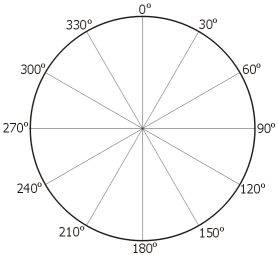### Longitude

Lines of longitude are also referred to as Meridians and Great Circles. Great Circles are defined as "any circle on the surface of a sphere, especially when the sphere represents the Earth, formed by the intersection of the surface with a plane passing through the center of the sphere." Any path with follows a great circle on the surface of the Earth will be the shortest possible distance between two points. All lines of longitude are great circles, as well as the Equator, which is a line of latitude.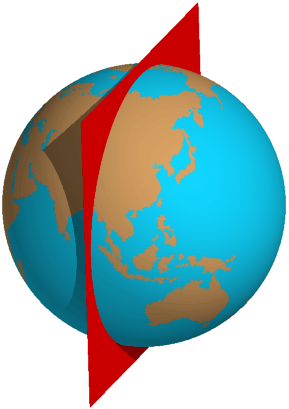Longitude lines are lines which run north-south on the globe. These lines all converge and meet at the North and South Poles. Because of this convergence the distance from one line of longitude to the next is not constant. As one moves towards the poles from the equator the east-west distance between lines decreases.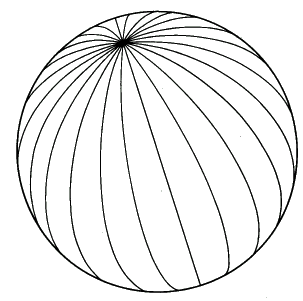Line of longitude are measured in degrees east or west of the Prime Meridian. This is easiest to understand when looking down on the Earth at the North Pole. From this perspective it is easy to see the angle that is formed between the Prime Meridian and the line of longitude. Because lines of longitude are measured east or west of the Prime Meridian it is important to place an "E" or "W" after the degrees. Half way around the globe from the Prime Meridian is 180°. This line of longitude (along with the Prime Meridian) does not have an "E" or "W" designation as 180° defines a single line. Note that this is not the case for 150° or any other line of longitude. Longitude measurements can only go from 0° to 180°.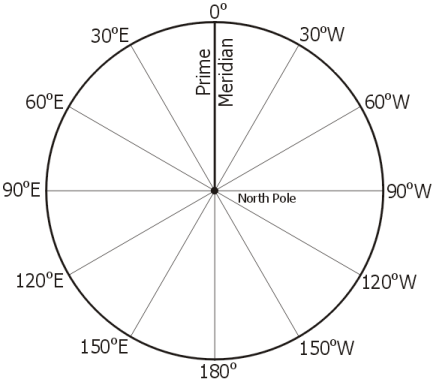### Latitude

Lines of latitude are a little bit harder to visualize how they are measured, but are still based on measuring angles. Latitude lines are lines which run east-west on the globe. They are also referred to as "Parallels". Unlike lines of longitudes, each line of latitude is parallel to every other line of latitude. In other words, two lines of latitude will never intersect or cross, and the distance between the two lines will always remain constant.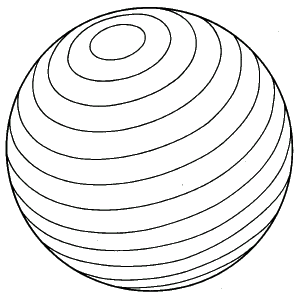Lines of latitude are measured in degrees north or south of the Equator, 0° latitude. In order to more easily understand how this angle is measured it is best to imagine that the Earth has been sliced in half along a great circle. The line connecting the North and South Poles is the Earth's axis of rotation. This is perpendicular, or at 90°, to the Equator. At the surface of the Earth, at a given latitude, draw a line from that location to the center of the Earth. The angle between this line and the Equator is the latitude measurement. The drawing below shows two examples - one for 30° N and one for 60° S. As with longitude, it is important to place the letter designations of "N" or "S" on the measurements. Only the Equator, 0°, does not have a north or south direction. Latitude measurements can only go from 0° to 90°.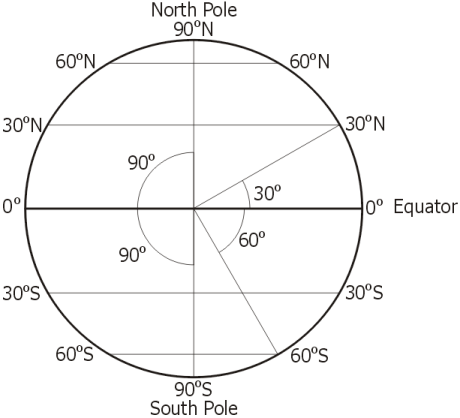### Determining Longitude and Latitude

The left diagram below shows a globe with the longitude and latitude lines on it. The Prime Meridian and Equator have been highlighted in red. The location at the point where these two lines meet would be designated as 0° Longitude, 0° Latitude. The red dot on the right hand diagram below would have a designation of 60°E Longitude, 30°N Latitude.Determine the Longitude and Latitude of the locations on the map below. Enter your answers in the spaces provided below, then grade your answers. Each Longitude and Latitude must be entered with a single space between the degrees and the N, S, E or W. For example, "10 W". The N, S, E and W must be entered as a capital letter.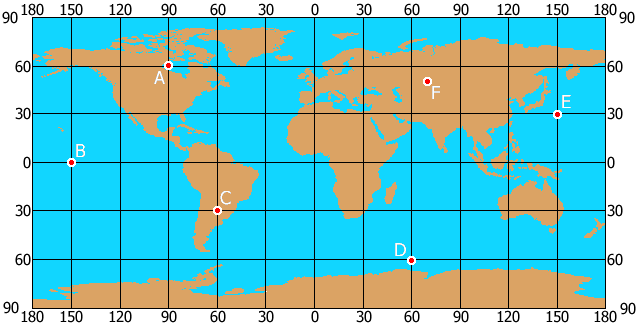Location Longitude Latitude
A
B
C
D
E
F